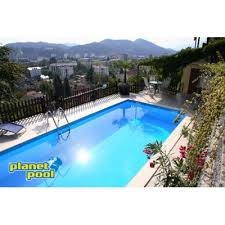# Three-quarters 6591

How many liters of water are in a cube-shaped pool with dimensions equal to 3 whole six meters b equals one full 9 meters and a depth of one total 5 meters if it is filled to only three-quarters of its volume?

V =  25650 l

### Step-by-step explanation:Did you find an error or inaccuracy? Feel free to write us. Thank you!

Tips for related online calculators
Need help calculating sum, simplifying, or multiplying fractions? Try our fraction calculator.
Do you know the volume and unit volume, and want to convert volume units?

#### Grade of the word problem:

We encourage you to watch this tutorial video on this math problem: